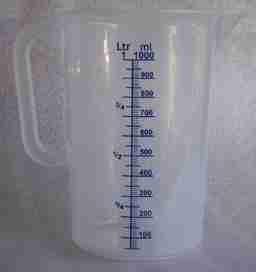# Definition of MillilitreA millilitre is a metric unit of volume that is equal to $\dfrac{1}{1000}$th of a litre.

We use millilitres to measure small volumes of liquid like doses of medicine or ingredients for cooking.

The abbreviation for millilitres is technically mL, but ml is commonly used.

One millitre is equal to one cubic centimetre.

For example,

• A metric teaspoon measures $5$ mL.
• An Australian metric tablespoon measures $20$ mL.
• Just about everyone else's metric tablespoon measures $15$ mL.
• A metric cup measures $250$ mL.
• A standard eye dropper has a volume of about $\dfrac{1}{20}$ mL.
• Measuring jugs are often marked in millilitres.

### Description

The aim of this dictionary is to provide definitions to common mathematical terms. Students learn a new math skill every week at school, sometimes just before they start a new skill, if they want to look at what a specific term means, this is where this dictionary will become handy and a go-to guide for a student.

### Audience

Year 1 to Year 12 students

### Learning Objectives

Learn common math terms starting with letter M

Author: Subject Coach
You must be logged in as Student to ask a Question.